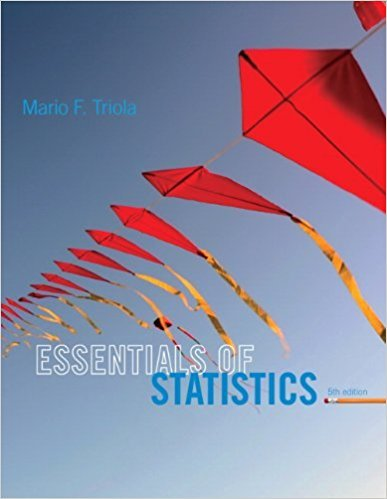×
×

# Solutions for Chapter 8.5: Testing a Claim About a Standard Deviation or Variance## Full solutions for Essentials of Statistics | 5th Edition

ISBN: 9780321924599Solutions for Chapter 8.5: Testing a Claim About a Standard Deviation or Variance

Solutions for Chapter 8.5
4 5 0 319 Reviews
24
4
##### ISBN: 9780321924599

Essentials of Statistics was written by and is associated to the ISBN: 9780321924599. Chapter 8.5: Testing a Claim About a Standard Deviation or Variance includes 20 full step-by-step solutions. This expansive textbook survival guide covers the following chapters and their solutions. Since 20 problems in chapter 8.5: Testing a Claim About a Standard Deviation or Variance have been answered, more than 14371 students have viewed full step-by-step solutions from this chapter. This textbook survival guide was created for the textbook: Essentials of Statistics, edition: 5.

Key Statistics Terms and definitions covered in this textbook
• Average

See Arithmetic mean.

• Binomial random variable

A discrete random variable that equals the number of successes in a ixed number of Bernoulli trials.

• Box plot (or box and whisker plot)

A graphical display of data in which the box contains the middle 50% of the data (the interquartile range) with the median dividing it, and the whiskers extend to the smallest and largest values (or some deined lower and upper limits).

• Combination.

A subset selected without replacement from a set used to determine the number of outcomes in events and sample spaces.

• Confounding

When a factorial experiment is run in blocks and the blocks are too small to contain a complete replicate of the experiment, one can run a fraction of the replicate in each block, but this results in losing information on some effects. These effects are linked with or confounded with the blocks. In general, when two factors are varied such that their individual effects cannot be determined separately, their effects are said to be confounded.

• Conidence coeficient

The probability 1?a associated with a conidence interval expressing the probability that the stated interval will contain the true parameter value.

• Conidence level

Another term for the conidence coeficient.

• Contingency table.

A tabular arrangement expressing the assignment of members of a data set according to two or more categories or classiication criteria

• Continuity correction.

A correction factor used to improve the approximation to binomial probabilities from a normal distribution.

• Continuous uniform random variable

A continuous random variable with range of a inite interval and a constant probability density function.

• Convolution

A method to derive the probability density function of the sum of two independent random variables from an integral (or sum) of probability density (or mass) functions.

• Covariance

A measure of association between two random variables obtained as the expected value of the product of the two random variables around their means; that is, Cov(X Y, ) [( )( )] =? ? E X Y ? ? X Y .

• Decision interval

A parameter in a tabular CUSUM algorithm that is determined from a trade-off between false alarms and the detection of assignable causes.

• Defect

Used in statistical quality control, a defect is a particular type of nonconformance to speciications or requirements. Sometimes defects are classiied into types, such as appearance defects and functional defects.

• Deming’s 14 points.

A management philosophy promoted by W. Edwards Deming that emphasizes the importance of change and quality

• Discrete random variable

A random variable with a inite (or countably ininite) range.

• Error mean square

The error sum of squares divided by its number of degrees of freedom.

• Error propagation

An analysis of how the variance of the random variable that represents that output of a system depends on the variances of the inputs. A formula exists when the output is a linear function of the inputs and the formula is simpliied if the inputs are assumed to be independent.

• Geometric mean.

The geometric mean of a set of n positive data values is the nth root of the product of the data values; that is, g x i n i n = ( ) = / w 1 1 .

• Harmonic mean

The harmonic mean of a set of data values is the reciprocal of the arithmetic mean of the reciprocals of the data values; that is, h n x i n i = ? ? ? ? ? = ? ? 1 1 1 1 g .

×